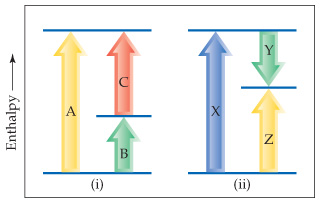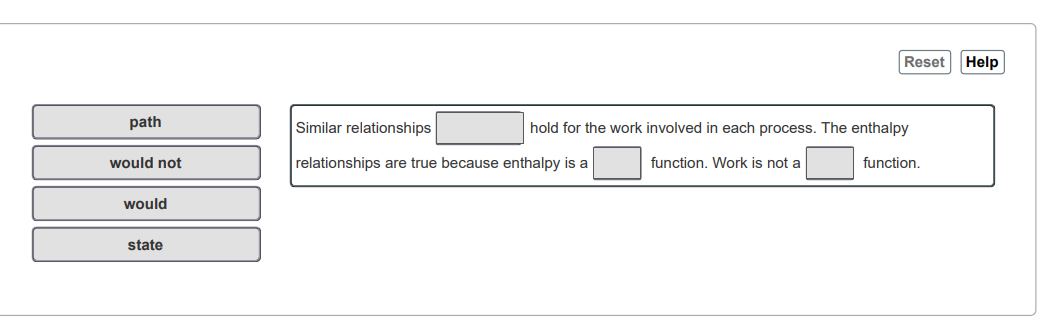# Problem: Consider the two diagrams below.Would similar relationships hold for the work involved in each process? Why?  Match the words in the left column to the appropriate blanks in the sentences on the right

###### FREE Expert Solution

Recall that:

$\mathbf{∆}{\mathbf{H}}_{\mathbf{rxn}}\mathbf{=}\mathbf{∆}{\mathbf{H}}_{\mathbf{f}\mathbf{,}\mathbf{products}}\mathbf{-}\mathbf{∆}{\mathbf{H}}_{\mathbf{f}\mathbf{,}\mathbf{reactants}}$

• Enthalpy → can be computed based on initial and final states
• State function → property of a system that does not depend on the path taken
• Work →  depends on the path taken###### Problem Details

Consider the two diagrams below.Would similar relationships hold for the work involved in each process? Why?  Match the words in the left column to the appropriate blanks in the sentences on the right